## Example Questions

### Example Question #1 : How To Find The Volume Of A Prism

A box's length is twice as long as its width. Its height is the sum of its length and its width. What is the volume of this box if its length is 10 units?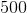units cubed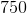units cubedunits cubedunits cubed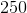units cubedunits cubed

Explanation:

The formula for the volume of a rectangular prism is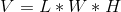, where "" is volume, "" is length, "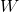" is width and "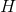" is height.

We know that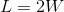and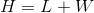. By rearranging, we get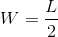. Substituting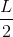into the volume equation forand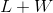into the same equation for, we get the following: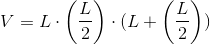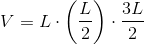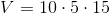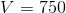units cubed

### Example Question #71 : Solid Geometry

A rectangular prism has the following dimensions:

Length: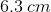Width:Height: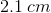Find the volume.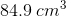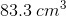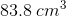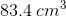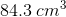Explanation:

Given that the dimensions are:,, andand that the volume of a rectangular prism can be given by the equation: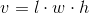, whereis length,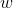is width, andis height, the volume can be simply solved for by substituting in the values.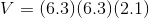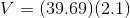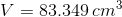This final value can be approximated to.

### Example Question #96 : Prisms

A rectangular box has two sides with the following lengths:andIf it possesses a volume of, what is the area of its largest side?

49

28

21

12

16

28

Explanation:

The volume of a rectangular prism is found using the following formula:If we substitute our known values, then we can solve for the missing side.Divide both sides of the equation by 12.We now know that the missing length equals 7 centimeters.

This means that the box can have sides with the following dimensions: 3cm by 4cm; 7cm by 3cm; or 7cm by 4cm. The greatest area of one side belongs to the one that is 7cm by 4cm.### Example Question #72 : Solid Geometry

Solve for the volume of a prism that is 4m by 3m by 8m.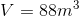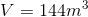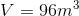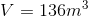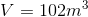Explanation:

The volume of the rectangleso we plug in our values and obtain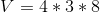.